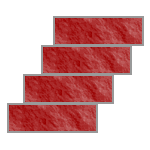Logic puzzles, riddles, math puzzles and brainteasers - pzzls.com

Vandaag is het 1 October 2022

# Brick tower - science puzzle

Difficulty:Rating: 2.6/5.0

Suppose you have an infinite number of bricks and no cement or another material to connect the bricks to each other. You would like to build a tower like the one in the figure. How many bricks do you need in order to build a tower in which the highest brick is shifted three bricks with respect to the lowest brick?# Explanation

If you build a tower from two bricks, the highest brick can maximally be shifted a half brick with respect to the lowest one. This is because if you shift the upper brick somewhat more, the center of mass of the upper brick is not longer right above the lowest brick but next to the lowest brick. Therefore in that case the tower will fall. The center of mass of this tower of two bricks lies at (distance till center of mass lowest brick + distance to center of mass upper brick) / (total mass) = (1/2 + 3/2) / 2 = 3/4 brick lengths from one side of the lowest brick.

You can put the tower of two bricks on top of a third brick. Then the center of mass of the tower of two bricks should be just along the corner of the lowest brick. That implies that the second lowest brick can be shifted maximally 1/4 brick with respect to the lowest brick. The maximal shift possible with a three brick tower is hence 1/2 + 1/4 = 3/4. The center of mass of the tower with three bricks lies at (1/2 + 3/4 + 5/4) / 3 = 5 / 6.

If would like to build a tower from four bricks, you put the three brick tower on a new lower brick. Since the center of mass of the three brick tower lies at 5/6 brick lengths, the three brick tower can be shifted at most 1/6. The total shift will be (1/6 + 1/4 + 1/2) = 11/12.

Continuing this reasoning shows that the maximal shift for a tower consisting of N bricks is equal to

[ 1 + 1/2 + 1/3 + 1/4 + 1/5 + ...... + 1/(N-1) ] / 2.

The question is to determine how many brick you need in order to build a tower with a shift of three bricks. You will get this shift if [1 + 1/2 + 1/3 + ... 1/(N-1) ] / 2 is larger than 3.

Using the formula gives that
if the number of bricks N = 227 then the maximal shift is 2.999981 and
if the number of bricks N = 228 then the maximal shift is 3.002183.

So you need at least 228 bricks in order to get a shift of three bricks.